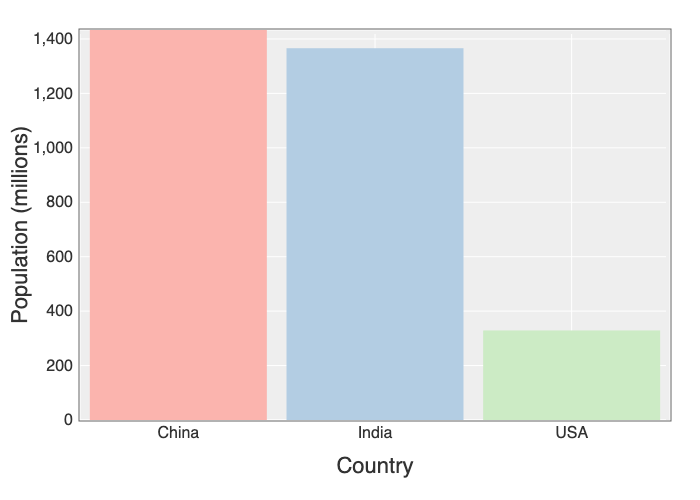# Daru: Pandas for RubyPhoto by Bruce Hong

2023 Update: Check out Polars Ruby as well.

NumPy and Pandas are two extremely popular libraries for machine learning in Python. Last post, we looked at Numo, a Ruby library similar to NumPy. As luck would have it, there’s a library similar to Pandas as well. It’s called Daru, and it’s the focus of this post.

## Overview

Daru is a data analysis library. Its core data structure is the data frame, which is similar to an in-memory database table. Data frames have rows and columns, and each column has a specific data type. Let’s create a data frame with the most populous countries:

``````df = Daru::DataFrame.new(
country: ["China", "India", "USA"],
population: [1433, 1366, 329] # in millions
)
``````

Population data from the United Nations, 2019

Here’s what it looks like:

``````     country population
0      China       1433
1      India       1366
2        USA        329
``````

You can get specific columns with:

``````df[:country]
df[:country, :population]
``````

Or specific rows with:

``````df.first(2)  # first 2 rows
df.last(2)   # last 2 rows
df.row    # 2nd row
df.row[1..2] # 2nd and 3rd row
``````

## Filtering, Sorting, and Grouping

Select countries with over 1 billion people.

``````df.where(df[:population] > 1000)
``````

For equality, use `eq` or `in`.

``````df.where(df[:country].eq("China"))
df.where(df[:country].in(["USA", "India"]))
``````

Negate a condition with `!`.

``````df.where(!df[:country].eq("India"))
``````

Combine operators with `&` (and) and `|` (or).

``````df.where(df[:country].eq("USA") | (df[:population] < 1400))
``````

Sort the data frame by a column with:

``````df.sort([:population])
df.sort([:country], ascending: [false])
``````

You can also group data and perform aggregations.

``````cities = Daru::DataFrame.new(
country: ["China", "China", "India"],
city: ["Shanghai", "Beijing", "Mumbai"]
)
cities.group_by([:country]).count
``````

## Combining Data Frames

There are a number of ways to combine data frames. You can add rows:

``````countries = Daru::DataFrame.new(
country: ["Indonesia", "Pakistan"],
population: [271, 217] # in millions
)
df.concat(countries)
``````

``````locations = Daru::DataFrame.new(
continent: ["Asia", "Asia", "North America"],
planet: ["Earth", "Earth", "Earth"]
)
df.merge(locations)
``````

You can also perform joins like in SQL.

``````cities = Daru::DataFrame.new(
country: ["China", "China", "India"],
city: ["Shanghai", "Beijing", "Mumbai"]
)
df.join(cities, how: :inner, on: [:country])
``````

Daru makes it easy to load data from a CSV file.

``````Daru::DataFrame.from_csv("countries.csv")
``````

After manipulating the data, you can save it back to a CSV file.

``````df.write_csv("countries_v2.csv")
``````

You can also load data directly from Active Record.

``````relation = Country.where("population > 100")
Daru::DataFrame.from_activerecord(relation)
``````

## Plotting

For plotting, use a Jupyter notebook with IRuby. Create a plot with:

``````df.plot type: :bar, x: :country, y: :population do |plot, diagram|
plot.x_label "Country"
plot.y_label "Population (millions)"
diagram.color(Nyaplot::Colors.Pastel1)
end
``````You can also create line charts, scatter plots, box plots, and histograms.

## Summary

You’ve now seen how to use Daru to:

• create data frames
• filter, sort, and group data
• combine data frames
• create plots

Try out Daru for your next analysis.

Published September 18, 2019

You might also enjoy

## Dokku on DigitalOcean

All code examples are public domain.
Use them however you’d like (licensed under CC0).